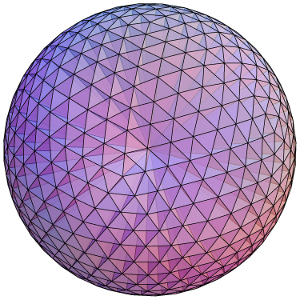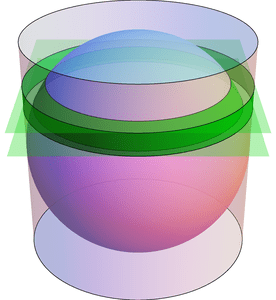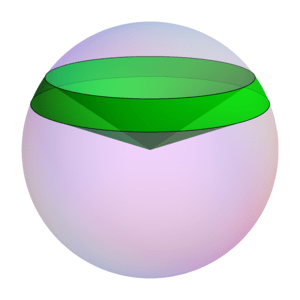# Spherical Surfaces and Hat Boxes

To round off our series on round objects (see the first and second posts), let’s compute the sphere’s surface area. We can compute this in the same way we related the area and circumference of a circle two weeks ago. Approximate the surface of the sphere with lots of small triangles, and connect these to the center of the sphere to create lots of triangular pyramids. Each pyramid has volume $$\frac{1}{3}(\text{area of base})(\text{height})$$, where the heights are all nearly $$r$$ and the base areas add to approximately the surface area. By using more and smaller triangles these approximations get better and better, so the volume of the sphere is $$\frac{4}{3}\pi r^3 = \frac{1}{3}(\text{surface area})\cdot r,$$ meaning the surface area is $$4\pi r^2$$. (This and previous arguments can be made precise with the modern language of integral calculus.)Dividing a sphere into many pyramids connected to the center allows us to relate the sphere's surface area and volume.

Here’s an elegant way to rephrase this result: The surface area of a sphere is equal to the area of the curved portion of a cylinder that exactly encloses the sphere. In fact, something very surprising happens here!:

Archimedes’ Hat-Box Theorem: If we draw any two horizontal planes as shown below, then the portions of the sphere and the cylinder between the two planes have the same surface area.Any two horizontal planes cut off a band on the sphere and another band on the enclosing cylinder. Archimedes' Hat-Box Theorem says that these bands have the same area. (The planes shown here have heights $$0.4\cdot r$$ and $$0.6\cdot r$$ above the equator.)

We can prove this with (all!) the methods in the last few posts; here’s a quick sketch. To compute the area of the “spherical band” (usually called a spherical zone), first consider the solid spherical sector formed by joining the spherical zone to the center:The Hat-Box theorem can be proved by relating the area of the spherical zone to the volume of this spherical sector.

By dividing this into lots of triangular pyramids as we did with the sphere above, we can compute the area of the spherical zone by instead computing the sector’s volume. This volume can be computed by breaking it into three parts: two cones and the spherical segment between the two planes (on the left of the next figure). Compute the volume of the spherical segment by comparing (via Cavalieri’s Principle) to the corresponding part of the vase (from the previous post), which can be expressed with just cylinders and cones.

1.Ross Anderson says: